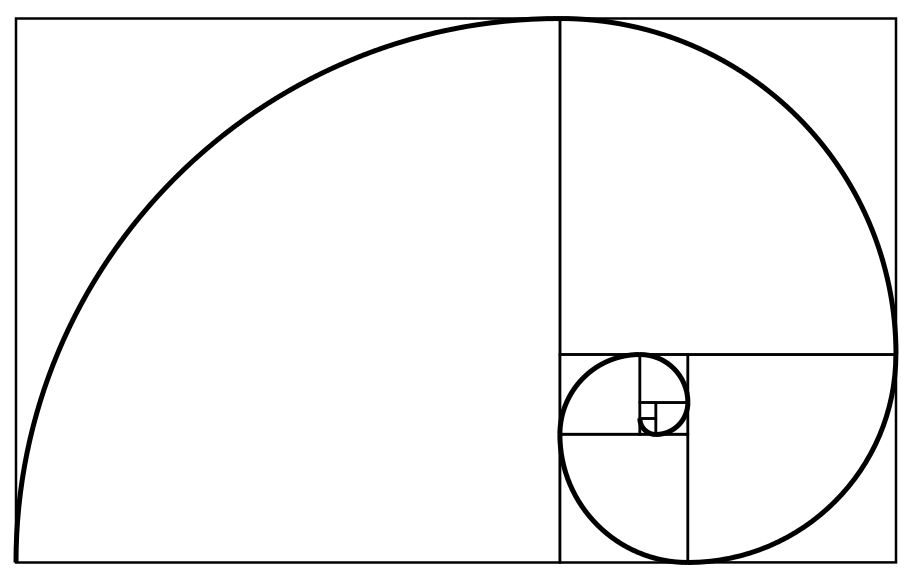Roots: the legacy of Fibonacci

More than spirals and rabbits, Fibonacci gave us something much more fundamental.Most initial thoughts when the name Fibonacci is mentioned centre around sequences, rabbits, nature and spirals. However, the Fibonacci legacy is much more fundamental to modern scientific studies, and without his influence, mathematics—as we know it—would not exist.The famous Fibonacci spiral

Leonardo, of the family of Bonacci, was born in Pisa, Italy, in around 1170. It wouldn’t be until the French mathematician, Édouard Lucas, wrote extensively about the $1, 1, 2, 3, 5, 8, \ldots$ sequence in 1877 that the “Fibonacci sequence” would become more well-known. Leonardo’s father was a successful merchant and customs officer, travelling around the Mediterranean with his family in tow.

Throughout his childhood, the young Fibonacci was encouraged by his father to study calculation. It was during a stay in Algeria that he became hooked on maths due to the “marvellous instruction” of his tutor.

In 1202, Leonardo published Liber Abaci—The Book of Calculation. This was the era of the Fourth Crusade, Genghis Khan and Eleanor of Aquitaine. There are no original copies of the book known to exist now, and only three copies of the second edition, printed in 1228, are left—these reside in Italy and the Vatican.

The book was an instruction manual for merchants and tradesmen. It contained worked problems with abstract ideas hidden within everyday examples of profit margins, currency conversions and tax: a 13th century “functional maths” course of great use to commercial tradesmen! One such problem is the following about birds:

“A certain man buys 30 birds which are partridges, pigeons, and
sparrows, for 30 denari
A partridge he buys for 3 denari,
a pigeon for 2 denari,
2 sparrows for 1 denaro,
namely 1 sparrow for 1/2 denaro.
It is sought how many birds he buys of each kind.”

The solution to this problem can be found at the end of this article.Engraving of Leonardo of Pisa

While it may seem unremarkable to our modern eyes, this bird problem beautifully illustrates Fibonacci’s legacy to mathematics: Liber Abaci introduced the western world to Hindu–Arabic numerals.

Leonardo’s book encourages and advocates the use of novem figuras indorum—nine Hindu figures—and tells the reader that with the numerals 9 to 1, along with the sign 0, any number may be written. He provides a translation from Roman numerals to his chosen figures.

Leonardo explains place value, intrinsic to maths today, and the radical idea that the position of each numeral shows what power of ten it represents. He goes to great lengths to describe how to calculate with these numerals, with examples of addition, subtraction, multiplication and division set out with increasing difficulty. He explains how to use fractions, ratio and proportion, and shows how to group digits in threes to make large numbers easier to read.

It’s interesting to note that, in keeping with the approach of the time, Leonardo describes 0 as a sign, rather than a numeral. He calls the sign “zephyr”, meaning “empty”, and groups it along with the operations rather than the digits.

Liber Abaci made Leonardo of Pisa famous. He attracted the attention of the Holy Roman Emperor, Frederick II, who was renowned for his thirst for knowledge. The two men wrote to each other for many years, and Fibonacci published more mathematical works, building on those first steps in Liber Abaci. Once the printing press was introduced to Europe in the 15th century, the use of the Hindu–Arabic number system spread even further.

Fibonacci’s legacy is visible to each and every one of us, every single day. It’s not in the number of petals on a flower, or spirals on a pine cone: it’s the numerals themselves. I for one think that’s an amazing gift to bestow.

Solution to the bird problem19th century statue of Leonardo Fibonacci in the Old Cemetery, Pisa

Let $x=\text{partridge}$, $y=\text{pigeon}$ and $z=\text{sparrow}$, so the problem gives us two equations:
\begin{align*}
x+y+z&=30,\\
3x+2y+\frac{1}{2} z&=30.
\end{align*}

We know that the values of $x$, $y$ and $z$ are actual birds, so must be positive whole numbers, and can’t be zero.

Doubling the second equation and subtracting the first to eliminate $z$ gives:
\begin{align*}
5x+3y=30.
\end{align*}

Now look at this last equation. The $5x$ and 30 both divide by 5, so it must also divide $3y$. Therefore, $y$ is a multiple of 5. But it can’t be 10 or larger else the last equation won’t work: $x$ cannot be zero. So
\begin{align*}